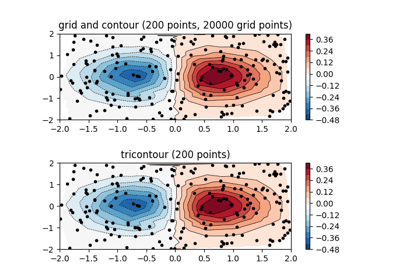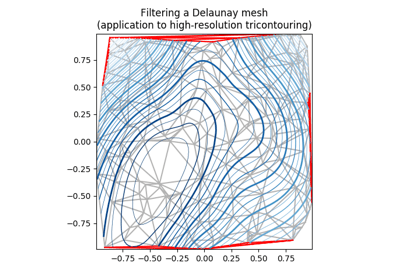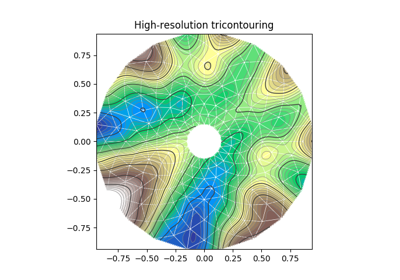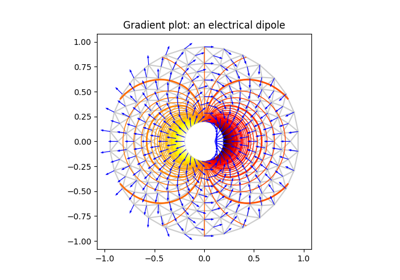/Matplotlib 3.0

# matplotlib.pyplot.tricontour

`matplotlib.pyplot.tricontour(*args, **kwargs)` [source]

Draw contours on an unstructured triangular grid. `tricontour()` and `tricontourf()` draw contour lines and filled contours, respectively. Except as noted, function signatures and return values are the same for both versions.

The triangulation can be specified in one of two ways; either:

```tricontour(triangulation, ...)
```

where triangulation is a `matplotlib.tri.Triangulation` object, or

```tricontour(x, y, ...)
tricontour(x, y, triangles, ...)
tricontour(x, y, triangles=triangles, ...)
```

in which case a Triangulation object will be created. See `Triangulation` for a explanation of these possibilities.

The remaining arguments may be:

```tricontour(..., Z)
```

where Z is the array of values to contour, one per point in the triangulation. The level values are chosen automatically.

```tricontour(..., Z, N)
```

contour up to N+1 automatically chosen contour levels (N intervals).

```tricontour(..., Z, V)
```

draw contour lines at the values specified in sequence V, which must be in increasing order.

```tricontourf(..., Z, V)
```

fill the (len(V)-1) regions between the values in V, which must be in increasing order.

```tricontour(Z, **kwargs)
```

Use keyword args to control colors, linewidth, origin, cmap ... see below for more details.

`C = tricontour(...)` returns a `TriContourSet` object.

Optional keyword arguments:

colors: [ None | string | (mpl_colors) ] If None, the colormap specified by cmap will be used.

If a string, like 'r' or 'red', all levels will be plotted in this color.

If a tuple of matplotlib color args (string, float, rgb, etc), different levels will be plotted in different colors in the order specified.

alpha: float The alpha blending value

cmap: [ None | Colormap ] A cm `Colormap` instance or None. If cmap is None and colors is None, a default Colormap is used.

norm: [ None | Normalize ] A `matplotlib.colors.Normalize` instance for scaling data values to colors. If norm is None and colors is None, the default linear scaling is used.

levels [level0, level1, ..., leveln] A list of floating point numbers indicating the level curves to draw, in increasing order; e.g., to draw just the zero contour pass `levels=`

origin: [ None | 'upper' | 'lower' | 'image' ] If None, the first value of Z will correspond to the lower left corner, location (0,0). If 'image', the rc value for `image.origin` will be used.

This keyword is not active if X and Y are specified in the call to contour.

extent: [ None | (x0,x1,y0,y1) ]

If origin is not None, then extent is interpreted as in `matplotlib.pyplot.imshow()`: it gives the outer pixel boundaries. In this case, the position of Z[0,0] is the center of the pixel, not a corner. If origin is None, then (x0, y0) is the position of Z[0,0], and (x1, y1) is the position of Z[-1,-1].

This keyword is not active if X and Y are specified in the call to contour.

locator: [ None | ticker.Locator subclass ] If locator is None, the default `MaxNLocator` is used. The locator is used to determine the contour levels if they are not given explicitly via the V argument.

extend: [ 'neither' | 'both' | 'min' | 'max' ] Unless this is 'neither', contour levels are automatically added to one or both ends of the range so that all data are included. These added ranges are then mapped to the special colormap values which default to the ends of the colormap range, but can be set via `matplotlib.colors.Colormap.set_under()` and `matplotlib.colors.Colormap.set_over()` methods.

xunits, yunits: [ None | registered units ] Override axis units by specifying an instance of a `matplotlib.units.ConversionInterface`.

tricontour-only keyword arguments:

linewidths: [ None | number | tuple of numbers ] If linewidths is None, defaults to rc:`lines.linewidth`.

If a number, all levels will be plotted with this linewidth.

If a tuple, different levels will be plotted with different linewidths in the order specified

linestyles: [ None | 'solid' | 'dashed' | 'dashdot' | 'dotted' ] If linestyles is None, the 'solid' is used.

linestyles can also be an iterable of the above strings specifying a set of linestyles to be used. If this iterable is shorter than the number of contour levels it will be repeated as necessary.

If contour is using a monochrome colormap and the contour level is less than 0, then the linestyle specified in `rcParams["contour.negative_linestyle"]` will be used.

tricontourf-only keyword arguments:

antialiased: bool enable antialiasing

Note: tricontourf fills intervals that are closed at the top; that is, for boundaries z1 and z2, the filled region is:

```z1 < z <= z2
```

There is one exception: if the lowest boundary coincides with the minimum value of the z array, then that minimum value will be included in the lowest interval.

## Examples using `matplotlib.pyplot.tricontour`Contour plot of irregularly spaced dataTricontour Smooth DelaunayTricontour Smooth User